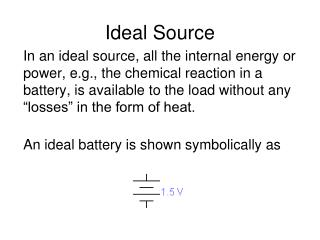DownloadDownload PresentationIdeal Source

# Ideal Source

Download Presentation## Ideal Source

- - - - - - - - - - - - - - - - - - - - - - - - - - - E N D - - - - - - - - - - - - - - - - - - - - - - - - - - -
##### Presentation Transcript

1. Ideal Source In an ideal source, all the internal energy or power, e.g., the chemical reaction in a battery, is available to the load without any “losses” in the form of heat. An ideal battery is shown symbolically as

2. An ideal voltage source (battery) in series with a load (light bulb): • Find the following: • The current through the bulb whose resistance is Rb = 2.5 Ω. • The power consumed by the light bulb in W. • The power supplied by the ideal battery in W.

3. Solutions The current drawn by the bulb is I = Vsource / Rbulb = 1.5 / 2.5 = 0.6 A The power consumed by the bulb is Pbulb= I2Rbulb = (0.6 A)2 2.5 = 0.9 W The power supplied by the ideal battery is Psource = V I = 1.5 (0.6) = 0.9 W From the law of the conservation of energy, Psource = Pbulb

4. Real Source • Real sources have an internal resistance. • This is not a visible resistor like you used in the lab, but it is a “model resistor” added to the circuit to analyze the source realistically. • The internal resistance causes energy loss (dissipated heat for example) and causes the source to provide less energy to the load.

5. Ideal source (battery) in series with a load (light bulb) Real source (battery with internal resistance) in series with a load (light bulb)

6. Repeat the previous exercise with a real 1.5 V battery source. Assume the battery’s internal resistance to be 0.3Ω. Find the following: Current through the bulb Power consumed by the bulb Power supplied by the ideal portion of the battery Power consumed by the battery’s internal resistance.

7. Req= Rinternal + Rb = 0.3 + 2.5 = 2.8 Ω • Current: I = 1.5 V / 2.8 Ω = 0.536 A • Power consumed by the bulb: Pbulb = I2Rbulb = (0.5357 A)2 2.5 Ω =0.717 W • Power provided by the ideal portion of the battery:Psource = V I = 1.5V(0.5357 A) =0.803 W • Power consumed by the battery’s internal resistance:Pinternal = I2 Rinternal = (0.5357 A)2 0.3 Ω =0.086 W

8. Ideal Source: I = 0.6 A Pbulb = 0.9 W Psource = 0.9 W Real Source: I = 0.536 A Pbulb = 0.717W Psource = 0.803W Pinternal = 0.086 W (dissipated as heat) Comparison with Previous Results Balance of power: Psource= Pbulb 0.9 W = 0.9 W Balance of power: Psource= Pinternal+ Pbulb 0.803W = 0.086W + 0.717W

9. Connection to Real Life • As batteries age, their internal resistance increases. • That means, even if you measure 1.5 V across the terminals with a meter, when you connect it to a load, it will provide a lower net voltage

10. In-Class Activity • Given this circuit where the real battery has been connected for a while. • Assume the bulb needs at least 0.4 A to light. • What will the internal resistance be when the bulb goes dark? • What voltage will be measured across the bulb at that time?

11. Question Can the bulb be replaced by a different bulb to maximize the power delivered to the bulb for a given battery voltage and internal resistance (Ri) ? In other words, if you replace the bulb with a symbolic resistance Rb as shown here, can you find the value of Rb for maximum bulb power?Start by expressing thepower absorbed by thebulb in terms of V and bothR’s.

12. Power Absorbed by Bulb • Pb = Ib * Vb • Ib =(in terms of Rb, Ri and Vs) • Vb = (in terms of Rb, Ri and Vs) • Therefore, Pb =

13. The power absorbed by the light bulb is given by where Pb = bulb power (W) Rb = bulb resistance (Ω) Ri = internal resistance (Ω) Vs = battery voltage (V)Is there a value of Rb that maximizes Pb?

14. Graphical ApproachIn-Class Activity Use Excel to generate a table of Pbas a function of Rb for V = 1.5 V and Ri = 0.3 Ω. Choose values of Rb from 0 Ω to 0.8 Ω in 0.05 Ω steps. Plot your data using the “Scatter Plot”

15. Vs = 1.5 V Ri = 0.3 Ω What is Pb for Rb= 0? What is Pbfor Rb → ∞ ? From the curve, identify what value of Rb makes Pb maximum.

16. AnalyticalApproach • In calculus you’ve learned about derivatives. • The first derivative of a function is a measure of its slope (rise/run). • When we take the derivative of our power function with respect to Rb, we find that the slope is zero at the maximum. • The value of Rb at that maximum will be Rb = Ri